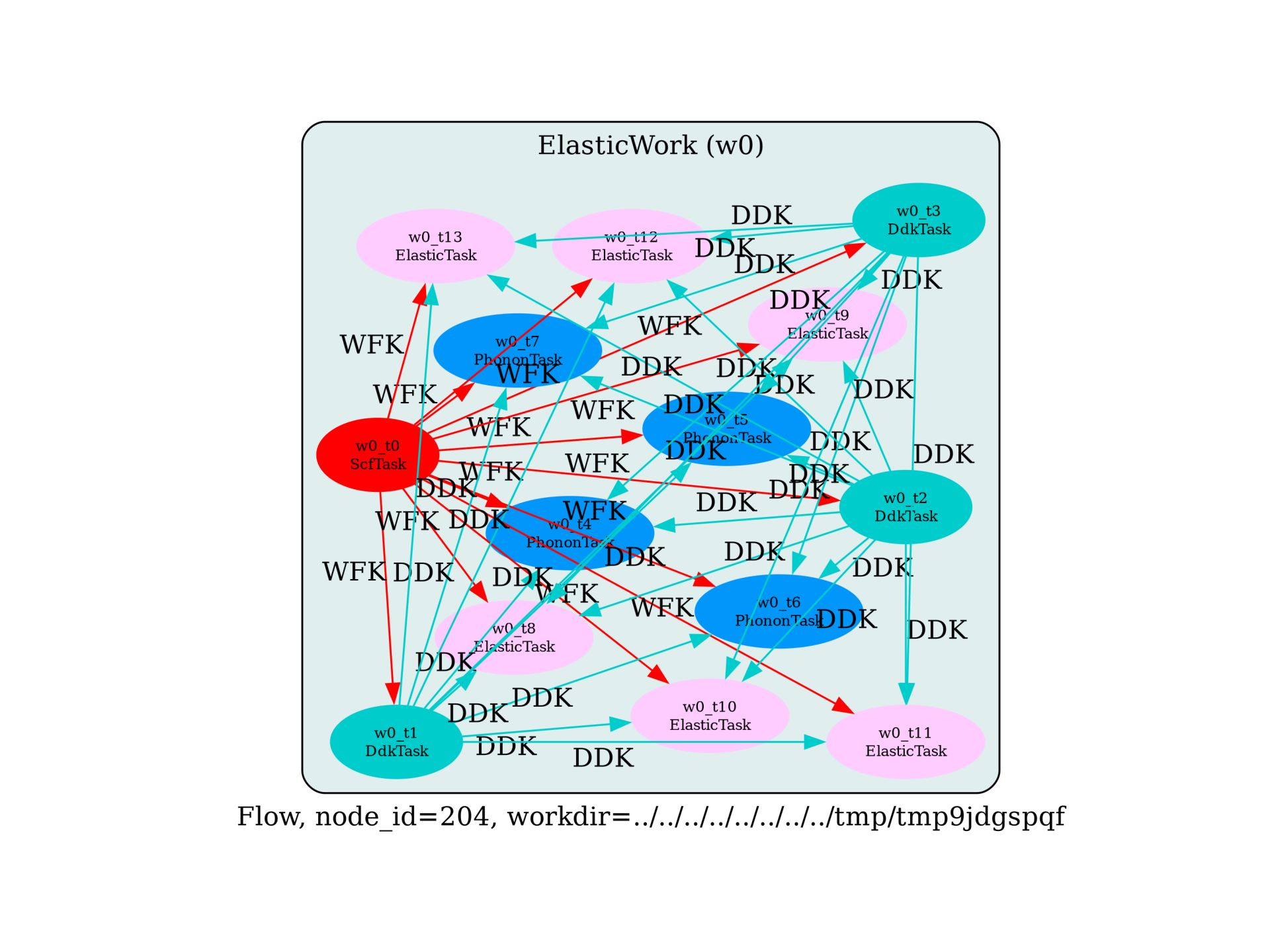# Flow for elastic constants and piezoelectric tensor with DFPT¶

This example shows how to use AbiPy to calculate physical properties related to strain for an insulator.

• the rigid-atom elastic tensor

• the rigid-atom piezoelectric tensor (insulators only)

• the internal strain tensor

• the atomic relaxation corrections to the elastic and piezoelectric tensor

Here we follow the discussion presented in in the the official tutorial

The DDB file with all the perturbations will be produced automatically at the end of the run and saved in flow_elastic/w0/outdata/out_DDB.

import sys
import os
import numpy as np
import abipy.abilab as abilab
import abipy.data as abidata

from abipy import flowtk

def make_scf_input(paral_kgb=0):
"""
This function constructs the input file for the GS calculation of
AlAs in hypothetical wurzite (hexagonal) structure.
In principle, the stucture should be relaxed before starting the calculation
"""

# Initialize structure. Use enough significant digits
# so that Abinit will recognize the correct spacegroup
# (Hexagonal and rhombohedral lattices are a bit problematic).
structure = abilab.Structure.from_abivars(
acell=[7.5389648144E+00, 7.5389648144E+00, 1.2277795374E+01],
natom=4,
ntypat=2,
rprim=[np.sqrt(0.75), 0.5, 0.0,
-np.sqrt(0.75), 0.5, 0.0,
0.0, 0.0, 1.0],
typat=[1, 1, 2, 2],
xred=[1/3, 2/3, 0,
2/3, 1/3, 1/2,
1/3, 2/3, 3.7608588373E-01,
2/3, 1/3, 8.7608588373E-01],
znucl=[13, 33],
)

pseudos = abidata.pseudos("13al.pspnc", "33as.pspnc")
gs_inp = abilab.AbinitInput(structure, pseudos=pseudos)

# Set other important variables (consistent with tutorial)
# Aall the other DFPT runs will inherit these parameters.
gs_inp.set_vars(
nband=8,
ecut=6.0,
ecutsm=0.5,        # Important when performing structural optimization
# with variable cell. All DFPT calculations should use
# the same value to be consistent.
ngkpt=[4, 4, 4],
nshiftk=1,
shiftk=[0.0, 0.0, 0.5],   # This choice preserves the hexagonal symmetry of the grid.
diemac=9.0,
nstep=40,
paral_kgb=paral_kgb,
tolvrs=1.0e-18,
)

return gs_inp

def build_flow(options):
"""
Create a Flow for phonon calculations. The flow has one work with:

- 4 Phonon Tasks (Gamma point)
- 6 Elastic tasks (3 uniaxial + 3 shear strain)

"""
# Working directory (default is the name of the script with '.py' removed and "run_" replaced by "flow_")
if not options.workdir:
options.workdir = os.path.basename(sys.argv).replace(".py", "").replace("run_", "flow_")

flow = flowtk.Flow(workdir=options.workdir)

# Build input for GS calculation and register the first work.
scf_input = make_scf_input()

elast_work = flowtk.ElasticWork.from_scf_input(scf_input, with_relaxed_ion=True, with_piezo=True)

flow.register_work(elast_work)

return flow

# This block generates the thumbnails in the AbiPy gallery.
# You can safely REMOVE this part if you are using this script for production runs.
__name__ = None
import tempfile
options = flowtk.build_flow_main_parser().parse_args(["-w", tempfile.mkdtemp()])
build_flow(options).graphviz_imshow()

@flowtk.flow_main
def main(options):
"""
This is our main function that will be invoked by the script.
flow_main is a decorator implementing the command line interface.
Command line args are stored in options.
"""
return build_flow(options)

if __name__ == "__main__":
sys.exit(main())Out:

/Users/gmatteo/git_repos/pymatgen/pymatgen/util/plotting.py:550: UserWarning: Matplotlib is currently using agg, which is a non-GUI backend, so cannot show the figure.
plt.show()


Run the script with:

run_elastic.py -s

then use:

abirun.py flow_elastic deps

to get the list of dependencies in the flow. Note, in particular, how the ElasticTasks depend on 3 DdkTasks and the initial ScfTask

<ElasticTask, node_id=340607, workdir=flow_elastic/w0/t13, qpt: (0, 0, 0), rfstrs: 2, rfdir: [0, 0, 1], irdddk: 1>
+--<DdkTask, node_id=340594, workdir=flow_elastic/w0/t1, qpt: (0, 0, 0), rfelfd: 2 rfdir: (1, 0, 0), irdddk: 0>
+--<DdkTask, node_id=340595, workdir=flow_elastic/w0/t2, qpt: (0, 0, 0), rfelfd: 2 rfdir: (0, 1, 0), irdddk: 0>
+--<DdkTask, node_id=340596, workdir=flow_elastic/w0/t3, qpt: (0, 0, 0), rfelfd: 2 rfdir: (0, 0, 1), irdddk: 0>


Use:

abiopen.py flow_elastic/w0/outdata/out_DDB -p

to print information about the DDB file. You should see that the DDB file contains:

Has (at least one) atomic pertubation: True
Has (at least one) electric-field perturbation: True
Has (at least one) Born effective charge: True
Has (all) strain terms: True
Has (all) internal strain terms: True
Has (all) piezoelectric terms: True


Now open the final DDB file with:

abiopen.py flow_elastic/w0/outdata/out_DDB

and invoke anaddb to compute the elastic and piezoelectric properties

In : edata = abifile.anaget_elastic()
In : print(edata)


Total running time of the script: ( 0 minutes 0.908 seconds)

Gallery generated by Sphinx-Gallery